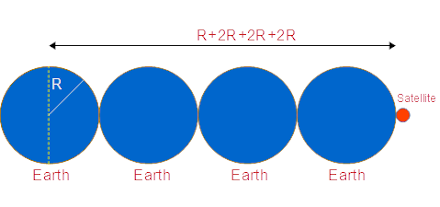# A satellite orbits Earth at a distance of 3 Earth above Earth's surface. (Earth mass equals 5.97...

## Question:

A satellite orbits Earth at a distance of 3 Earth above Earth's surface. (Earth mass equals 5.97 X 10{eq}^{24} {/eq}kg, earths equatorial radius equals 6.38 X 10{eq}^6 {/eq}m) How many Earth radii is the satellite form Earths center?

## The Earth:

Earth is a planet of our solar system.

The mass of the Earth is;

{eq}M_e = 5.97\times 10^{24} \ kg {/eq}

The radius of the Earth is;

{eq}R_e = 6.38 \times 10^6 \ m {/eq}

The position of the satellite is shown in the figure below.Given

• Radius of the earth is {eq}R = 6.38\times 10 ^6\ m {/eq}

Let

• Mass of the earth is {eq}M_e {/eq}
• Mass of the satellite is {eq}m {/eq}

According to the question orbiting distance (d) of the satellite above the surface of the earth is

{eq}\begin{align} d&= R+2R+2R+2R\\ &=7R\\ &=7\times (6.38\times 10 ^6\ m)\\ &=4.466\times 10^7\ m\\ \end{align} {/eq}